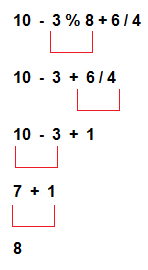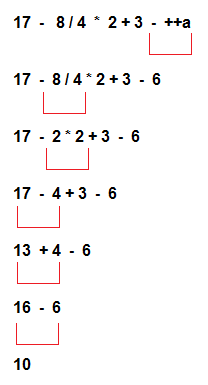# Expression evaluation in C

## Expression Evaluation in C

In c language expression evaluation is mainly depends on priority and associativity. An expression is a sequence of operands and operators that reduces to a single value. For example, the expression, 10+15 reduces to the value of 25.
An expression is a combination of variables constants and operators written according to the syntax of C language. every expression results in some value of a certain type that can be assigned to a variable.

## Priority

This represents the evaluation of expression starts from "what" operator.

## Associativity

It represents which operator should be evaluated first if an expression is containing more than one operator with same priority.

OperatorPriorityAssociativity
{}, (), []1Left to right
++, --, !2Right to left
*, /, %3Left to right
+, -4Left to right
<, <=, >, >=, ==, !=5Left to right
&&6Left to right
||7Left to right
?:8Right to left
=, +=, -=, *=, /=, %=9Right to left

### Example 1:### Example 2:### Types of Expression Evaluation in C

Based on the operators and operators used in the expression, they are divided into several types. Types of Expression Evaluation in C are:

• Integer expressions – expressions which contains integers and operators
• Real expressions – expressions which contains floating point values and operators
• Arithmetic expressions – expressions which contain operands and arithmetic operators
• Mixed mode arithmetic expressions – expressions which contain both integer and real operands
• Relational expressions – expressions which contain relational operators and operands
• Logical expressions – expressions which contain logical operators and operands
• Assignment expressions and so on… – expressions which contain assignment operators and operands